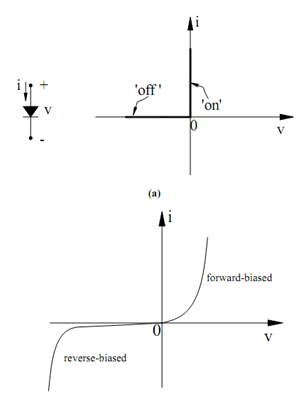## Models of Ideal and Practical Diodes Assignment Help

Assignment Help: >> Rectifier Circuits - Models of Ideal and Practical Diodes

Models of Ideal and Practical Diodes:

Figure (a) illustrates the v-i characteristics of an ideal diode which is also the characteristics of a switch. We shall use these characteristics to approximate those of a practical diode(b)

Figure: (a) Symbol and Current Flow Direction of an Ideal Diode. The On-off States of Ideal Diode Mimic those of an On-off Switch; and (b) A Practical Diode Characteristics

The v-i characteristics of a practical diode are illustrated in Figure (b). Note down that although generally, the ideal diode is a good approximation to a practical diode, the following differences can be noted :

1.      Under forward bias conditions, a diode takes a finite forward voltage of about 0.7 V for a practical diode to conduct. This voltage drop is termed as 'cut-in' voltage of the diode and is maintained throughout conduction.

2.      The maximum forward current is restricted by the heat-dissipation ability of the diode; for the IN 4002, for instance, maximum forward current is approximate 1 A.

3.      There is a small but finite reverse current which can not be critical in a practical circuit, as this is in the nano-ampere range.

4.      Every diode has a maximum reverse voltage that may not be exceeded. If the diode breaks down, a large reverse current pass that causes the diode to overheat and be permanently damaged. For the IN 4002, the reverse breakdown voltage is 100 V. If there is a possibility that the voltage across the diode can exceed the breakdown voltage, it is advisable that a diode with a larger breakdown voltage should be chosen.#### Assured A++ Grade

Get guaranteed satisfaction & time on delivery in every assignment order you paid with us! We ensure premium quality solution document along with free turntin report!

All rights reserved! Copyrights ©2019-2020 ExpertsMind IT Educational Pvt Ltd